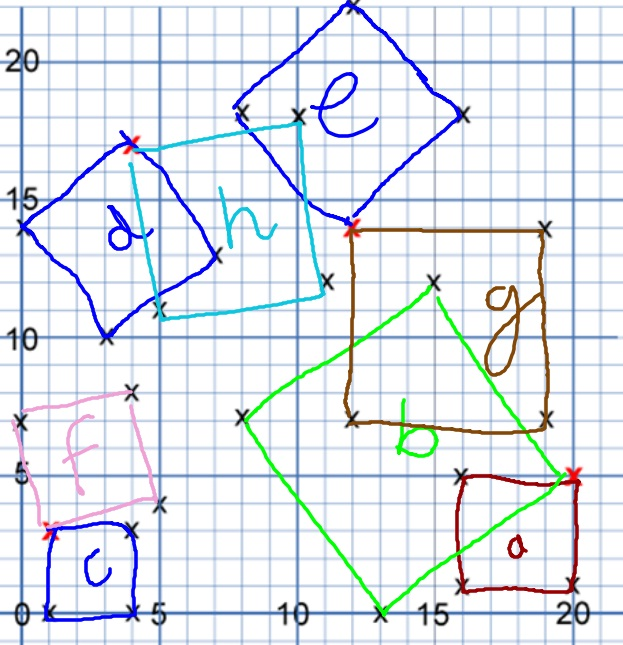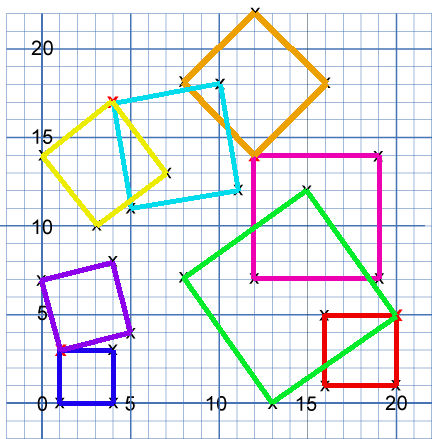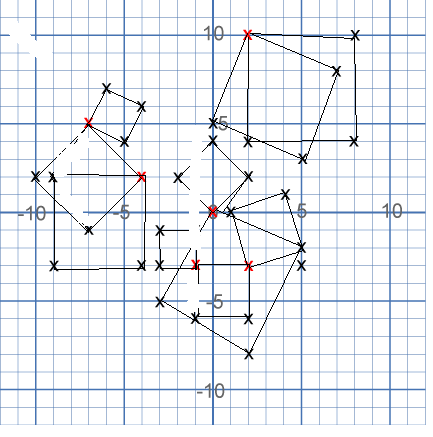#### You may also like### Square Areas

Can you work out the area of the inner square and give an explanation of how you did it?### Dissect

What is the minimum number of squares a 13 by 13 square can be dissected into?### 2001 Spatial Oddity

With one cut a piece of card 16 cm by 9 cm can be made into two pieces which can be rearranged to form a square 12 cm by 12 cm. Explain how this can be done.

# Hidden Squares

##### Age 11 to 14Challenge Level

Lots of school groups handed in excellent solutions to this problem.
Well done particularly to Ramu and Atasha from Brookland Primary School for their answers:

Square 1 - (1,0) , (1,3) , (4,0) , (4,3)
Square 2 - (1,3) , (0,7) , (5,4) , (4,8)
Square 3 - (20,1) , (16,1) , (16, 5) , (20,5)
Square 4 - (0, 13) , (8,7) , (20,5) , (15,12)
Square 5 - (12,7) , (12,14) , (19,7) , (19,14)
Square 6 - (12,14) , (8,18) , (12,22) , (16,18)
Sqaure 7 - (5,11) , (11,12) , (10,18) , (4,17)
Sqaure 8 - (0,14) , (3,10) , (7,13) , (4,17)

The Year 4s from Bradfield Dungworth sent in their picture:
AsAs did the Year 6s from Wingrave C of E:For the second part, Harjun sent in this image to show us where the ten squares are:There is just one square which you've mis-drawn a line in I think, Harjun. Can you see which one it is? Akalya and Michelle from Devonshire Primary School and Esther sent in the coordinates of the 10 squares so we can check that way, too:

1.(2,-3) (-1,-3) (-1,-6) (2,-6)
2.(0,0) (5,-3) (2,-8) (-3,-5)
3.(0,0) (2,2) (0,4) (-2,2)
4.(1,0) (4,1) (5,-2) (2,-3)
5.(2,10) (8,10) (8,4) (2,4)
6.(2,10) (7,8) (5,3) (0,5)
7.(-1,-1) (-1,-3) (-3,-3) (-3,-1)
8.(-4,2) (-9,2) (-9,-3) (-4,-3)
9.(-7,5) (-10,2) (-7,-1) (-4,2)
10.(-7,5) (-5,4) (-4,6) (-6,7)

Thank you to all of you.